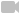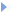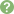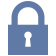Updated: 6/17/2018

# Plasma Membrane

0%
Topic
Review Topic
0
0
N/A
N/A
Questions
1
0
0
100%
0%
Evidence
1
0
0
0%
0%
Videos / Pods
3
Topic
 Overview Structurebilayer of phospholipids asymmetrical in respect to intracellular and extracellular faces "fluid mosaic" composition cholesterol ~50% adds thermal stability to membrane i.e. ↑ melting temperature ↓ membrane flexibility amount in plasma membrane tightly regulated phospholipids ~50% sphingolipids fatty acid chain attached to a sphingosine disorders of sphingolipid metabolism Fabry's disease Gaucher's disease see Lysosome topicglycolipids proteins pumps move substances against their concentration gradient requires energy e.g. Na+-K+ ATPase transmembrane pump ATP binding-site accessible from cytoplasm process 3 Na+ bind pump intracellularly ATP binds and phosphorylates the pump pump changes conformation which releases 3 Na+ extracellularly 2 K+ bind pump extracellularly phosphate ion removed pump changes conformation which releases 2 K+ intracellularly 3 Na+ bind pump intracellularly (repeat) net: each ATP results in 3 Na+ out and 2 K+ in pharmacological importance cardiac glycosides (digoxin and digitoxin) mechanism of action inhibits pump depolarization of cell membrane ↑ intracellular [Na+] ↓ Na+ gradient required for Na+/Ca2+ exchange ↑ [Ca2+] intracellularly ↑ cardiac contractility ouabain inhibits by binding to K+ site similar response to cardiac glycosides channels move substances down their concentration gradient 3 types ungated always open e.g. K+-channel voltage-gated open in response to changes in membrane voltage e.g. found in excitable tissue ligand-gatedopen in response to a ligand e.g. post-synaptic membrane receptors

 Function Function selective permeability controls an intracellular environment distinct from extracellular environment signalling localization of enzymes to promote or inhibit interaction Membrane Physiology Electrochemical potential determined by conductance (G) ability of ions to move across a membrane controlled by opening and closing channels ↑ channels = ↑ conductance net force combination of concentration force concentration difference of a substance across a membrane electrostatic force attraction of unlike charges repulsion of like charges Equilibrium potential defined as the electrical potential across a membrane that would prevent the diffusion of a substance via its concentration force for a given concentration difference across a membrane measured in millivolts (mV) for a single substance calculated by Nernst equation Ex+ = 60/Z log( [X+]extracell / [X+]intracell ) Z = absolute value of ionic charge K+, Cl-, Na+ = 1, Ca2+ = 2 Answers the question: what is the voltage that exists across a membrane when a certain ion is at its equilibrium Another way of thinking of this: what is the voltage required so that there will be no net flow of a certain ion? For example: -80 mV is the Nernst potential for potassium this means that if the inside of the cell was -80 mV, K+ would not leave or enter the cell The -80 mV of the cell pulling K+ in is equal to the concentration gradient that wants to pull K+ out (remember that K+ is low outside the cell and wants to travel down its ion gradient) If the voltage became -81 mV then K+ would want to travel into the cell as this negative charge would overpower the drive for K+ to travel down its concentration gradient out of the cell If the voltage was -79 mV then K+ would leave the cell as the concentration gradient pulling K+ out is greater than the negative voltage attracting/holding K+ in does NOT determine rate of ionic diffusion → only whether diffusion is favorable Resting membrane potential (Emem) equilibrium potential of most cells = -90 mV calculated by sum of individual membrane potentials for all permeable ions proportional to their conductances for ions X+, Y+, and Z- Emem = Gx(Ex+) + Gy(Ey+) + Gz(Ez-) note: the closer the resting membrane potential is to the equillibrium potential of an individual ion, the greater the membrane conductance is for that ion when Emem = Ex+ , there is no net movement of ions and net force = 0 example Emem = -77 mV EK+ = -95 mV is diffusion of K+ across this membrane favorable? Yes, given open channels (G) to K+ it will diffuse until Emem = -95 mV inside cells (as compared to extracellular environment) ↑ K+ EK+ = -95 mV G is high for K+→ changes in [K+]extracellular will have a large impact on Emem hyper/hypokalemia very dangerous clinically ↑ G will hyperpolarize cell efflux from cell ↓ Na+ ENa+ = +45 mV G is low for Na+ → changes in [Na+]extracellular will NOT have a large impact on Emem ↑ G will depolarize cell influx into cell ↓ Cl- ECl- = -90 mV since in most cells Emem = -90 mV → Cl- is at equilibrium and will not diffuse